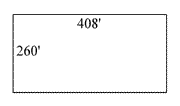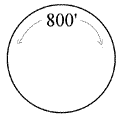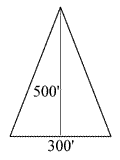# Managing Nuisance Aquatic Plants

## Estimating the Size of Your Pond

For some methods of vegetation control, you'll need to determine the surface acreage and/or the volume of water in your pond. This information will help:

### Basic Terms and Conversion Factors

• 1 acre = 43,560 square feet
• 1 acre-foot = 1 surface acre 1 foot deep, or 43,560 cubic feet
• Total volume in acre-feet = surface area (acres) x average depth (feet)
• 1 part per million (ppm) of herbicide = 2.7 pounds per acre-foot of water

### Calculating Surface AcreageRectangular pond - multiply length by width and divide by 43,560 (the number of square feet in one acre). In the diagram shown here, the equation would be:

260 feet x 408 feet = 106,080 square feet
106,080/43,560 = 2.44 surface acres.If the pond is circular, the distance circumference of the pond (distance around the shoreline) should be measured in feet. That number should be multiplied by itself and then divided by 547,390. If the pond's circumference is 800 feet, the equation would be:

800 feet x 800 feet = 640,000
640,000/547,390 = 1.17 acresIf the pond is triangular, the base and the height should be measured. Multiply those two numbers, take half of the resulting amount, and divide by 43,560. If the triangle is 300 feet at the base and 500 feet high, the equation would be:

(300 feet x 500 feet)/2 = 75,000 square feet
75,000/43,560 = 1.72 acres

### Calculating Average Depth

Make a series of soundings by dropping a weighted line to the bottom in straight rows across the pond 30 to 50 feet apart. Add the depth measurements together and divide by the number of soundings. Begin and end each row with the zero measurement at the shoreline and include these in the total number of soundings made.